3.9

# Recommended reading - A simple climate model with ocean heat uptake

## A simple model of the climate system

By using the concepts of radiative forcings, climate feedbacks and ocean heat uptake we can make a conceptual model that make use of the above three concepts to demonstrate how temperature changes are influenced by the three parameters.

Mathematically it contains two equations: One for the upper ocean and one for the deep ocean. The two are connected through a heat transfer parameter ($\gamma$) which transfer heat from the upper to the deeper ocean.

The upper ocean is given as:

$C_{mix} \frac{d \triangle T_s}{dt}= \triangle Q + \lambda \triangle T_s + \gamma ( \triangle T_s - \triangle T_o)$

where s $\triangle T_{s}$ (K) is the temperature change in the upper ocean, $\triangle Q$ ( W/m$^2$) is the radiative forcing, $\lambda (K/(W/m^2)$ is the sum of the feedback factors (see week two article on feedbacks), $\triangle T_o$ is the deep ocean temperature change and $\gamma$ the heat transfer parameter that transfer heat from the upper to the deeper ocean. $C_{mix}$ is the effective heat capacity of the upper ocean that will respond quite fast to the radiative forcing (a few decades) due to efficient wind induced mixing.

The deep ocean can only change its temperature by receiving more or less heat from the upper ocean:

$C_{deep} \frac{d \triangle T_o}{dt} = - \gamma (\triangle T_s - \triangle T_o)$

$C_{deep}$ is the effective heat capacity of the deep ocean (note that is this simplification we have assumed that the effective heat capacity will not change with time).

In the model we neglect land areas. Thus, $\triangle T_s$ should be seen as being representative for near surface ocean temperature changes.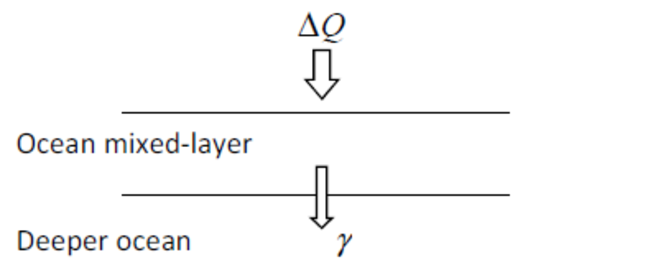Figure: Schematic of the simple model forced with a given radiative forcing ($\triangle Q$) and where the transport of heat into the deeper ocean is given by a heat transfer parameter $\gamma$ .

In order to use the model we will need values for the radiative forcing ($\triangle Q$), the feedbacks ($\lambda$) and the upper to deep ocean heat transfer parameter ($\gamma$).
Previously in the course we have gone through typical values for the radiative forcing ($\triangle Q$) and feedback parameters ($\lambda$) , but in order to use the above equations we need values for the ocean heat transfer parameter ($\gamma$). An approximate measure of the downward heat loss into the deep oceans is the ocean heat uptake efficiency (W/(m$^2$K)) which is a measure of the rate at which heat storage by the global ocean increases as global mean surface temperature rises. It is a useful parameter for climate change experiments where the radiative forcing is changing monotonically (alway increasing or decreasing), as it can be compared with the climate feedback parameters (see week 2 article) to investigate the relative importance of climate response and ocean heat uptake in determining the speed of climate change. It can be estimated from model experiments as the ratio of the rate of increase of ocean heat content to the global mean surface air temperature change. The table below gives typical values for the ocean heat uptake efficiency. A large negative number indicates a large heat loss into the deep oceans.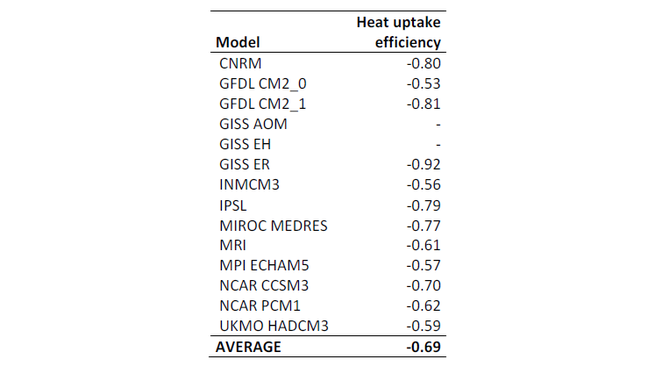Table: Tabulated values of the ocean heat uptake efficiency [W/(m$^2$K)] (from Dufresne and Bony, 2008)

As the mixed layer equilibrate within a few decades to a new radiative forcing, using the ocean heat uptake efficiency as a measure for the upper to deep ocean heat transfer parameter ($\gamma$) means that our model should be ok for timescales longer than a few decades.

Note that this model is only applicable to a transient climate, not a climate in equilibrium. Setting $\gamma$ to zero and assuming equilibrium ($d \triangle T_o$/dt is zero) the model reduces to the equilibrium temperature response given in the feedback article in week 2.

$\triangle T_{eq}= - \frac{1}{ \lambda } \triangle Q$

### Example: Calculating the transient change in temperature due a 1% per year change in CO$_2$

From the radiative forcing reading material for week 1 (task 1.11) the radiative forcing of CO$_2$ can be approximate as:

$RF_{CO_2} = \alpha$ln$(C/C_0)$

where $\alpha$ = 5.35 , $C_0$ is the reference CO$_2$ concentration (usually takes as 280 ppm) and C the CO$_2$ concentration. C and C$_0$ must be given in ppm (parts per million).

From the feedback article in week 2 (task 2.8) we get a number for the total feedback $W/(m^2K)$ and from the table above the effective ocean heat uptake is set to -0.69 $W/(m^2K)$. The transient temperature change is seen in the blue curve in figure c below. At doubling of CO$_2$ the temperature change is 1.8°C. If we perform the same simulation, but this time with the effective ocean heat uptake reduced to half the values (-0.35 $W/(m^2K)$), less heat will go into the deeper ocean and the near surface temperature will increases more. At time of doubling of CO$_2$ we get a temperature change of 2.1°C (red curve in figure c below). This illustrates the importance of effective ocean heat uptake in slowing down the speed of the temperature changes. .

a.)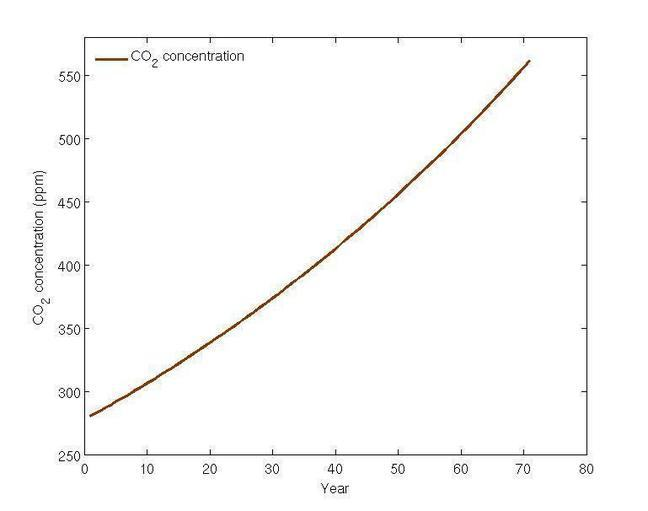b.)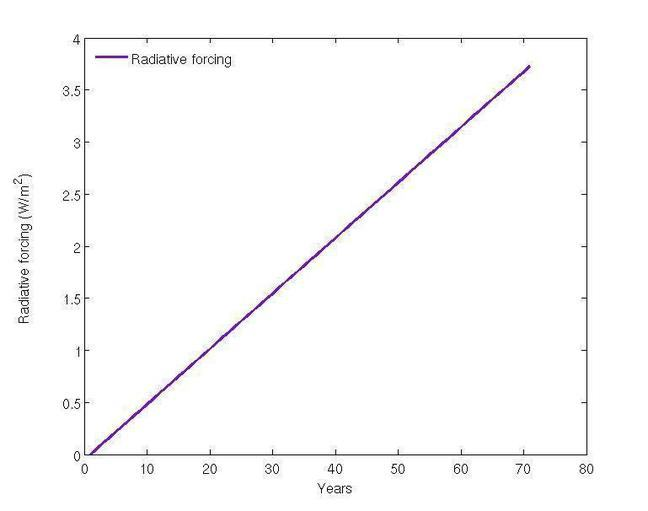c.)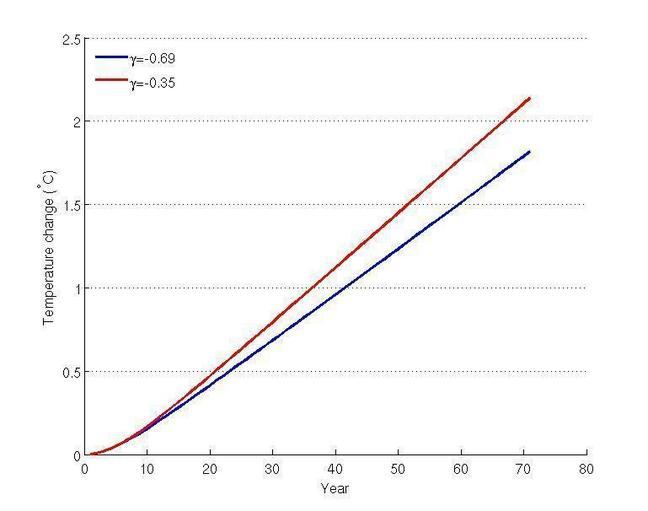Figure: The change in CO$_2$ concentrations given a 1% per year change in CO$_2$ (a), the radiative forcing (b) and the temperature response (c) with two different effective ocean heat uptakes.